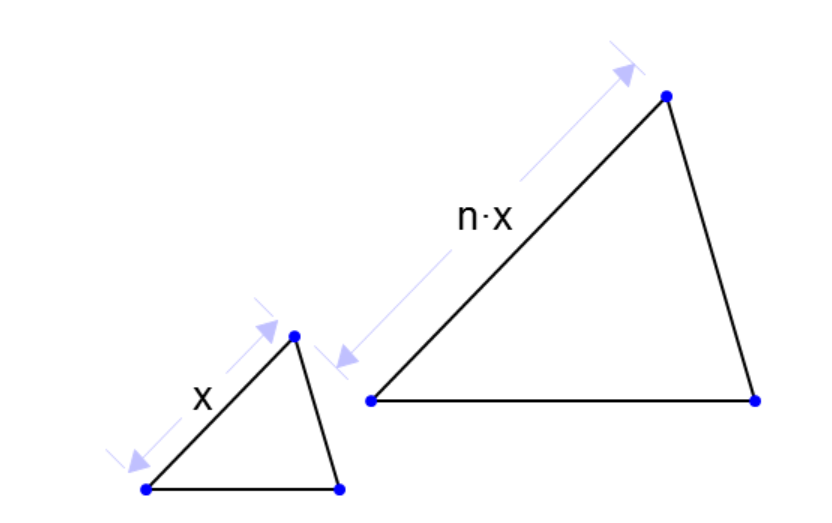## Proportions review

### What is a ratio?

A ratio is teh comparison of the quantity of two properties or items

### What is a proportion?

Proportion is an equation that states that two ratios are equal for instance a/b = c/d

### How do I convert from one unit to another using proportions?

Make a proportion quation using The conversion ratio and The a conversion statement for the unknown For example To convert 2 feet into inches make two statements 1 foot =12 inches 2 feet = x write them as ratios 1 foot/12 inches = 2 feets/x cross multiply x = 24 feet

### How to find arc length, curcumference or angle using proportion

x degrees/360 degrees = arc length /circumference For example: If the circumference of a circle is 24cm, what is the central angle that subtends an arc AB whose length is 4cm? x degrees/360 = 4cm/24cm cross multiply 24 x = 1440 divide both sides by 24 x = 60 degrees

### How to find side length of similar trianglesThe scale factor above is n This means that each side on the smaller triangle is multiplied by n to get the corresponding side length of the larger triangle. If the side lenght of the base of the small traingle is 4cm, and n is 2. then x/2x = 4/a cross multiply ax = 8x a = 8cm

### How to solve problems where two variables x and y are directly proportional### How to solve problems where the two variable x and y are inversely proportional